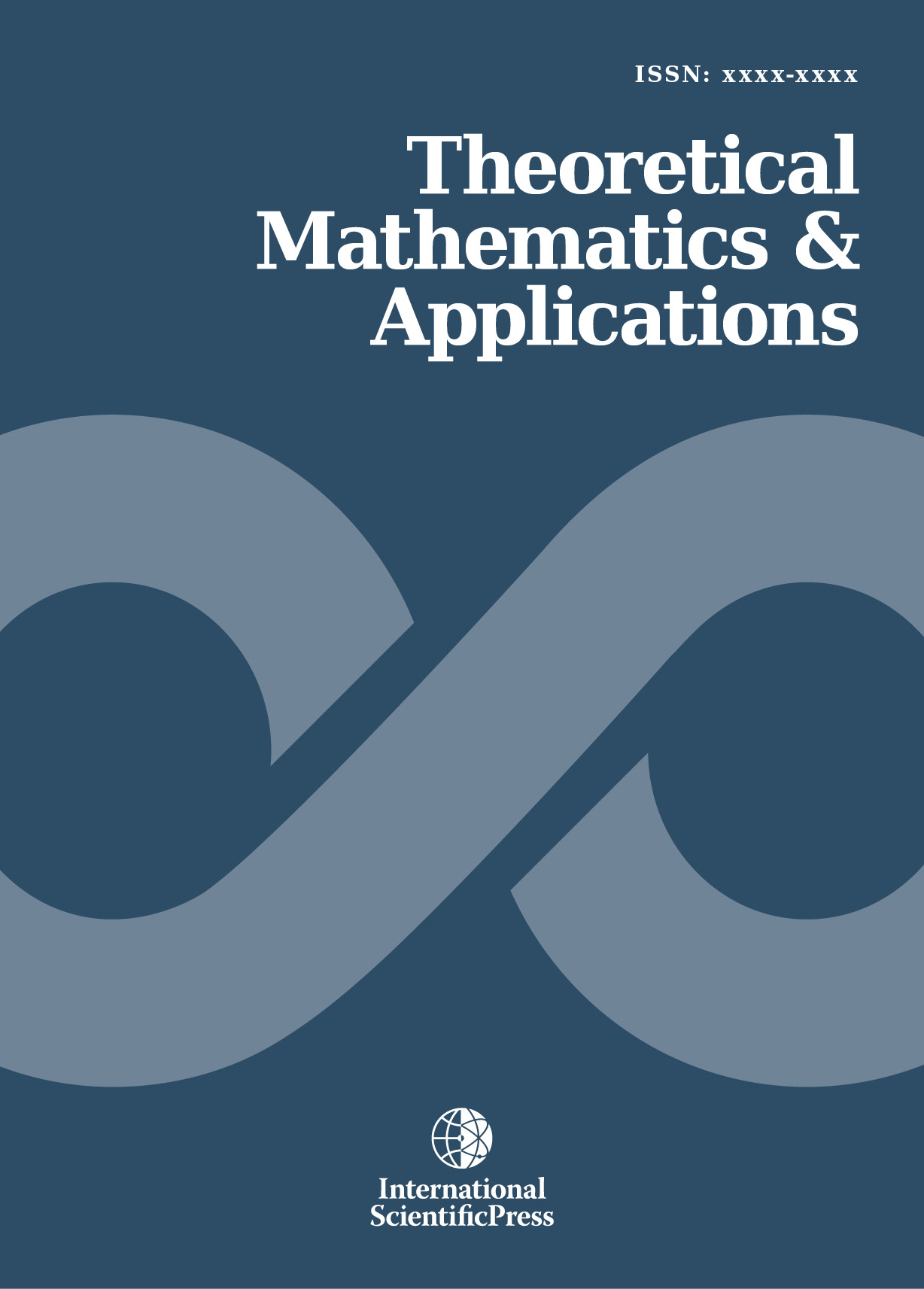# Theoretical Mathematics & Applications

#### Alternative Approach to Estimating the Parameters of Continuous Probability Distribution: Use of Order Statistics

•[ Download ]
• Times downloaded: 1063
• Abstract

Application of the method of moments for the parametric distribution is common in the construction of a suitable parametric distribution. However, moment method of parameter estimation does not produce good results. An alternative approach when constructing an appropriate parametric distribution for the considered data file is to use the so-called order statistics. This paper deals with the use of order statistics as the methods of L-moments and TL-moments of parameter estimation. L-moments have some theoretical advantages over conventional moments. L-moments have been introduced as a robust alternative to classical moments of probability distributions. However, L-moments and their estimations lack some robust features that belong to the TL-moments. TL-moments represent an alternative robust version of L-moments, which are called trimmed L-moments. This paper deals with the use of L-moments and TL-moments in the construction of models of wage distribution. Three-parametric lognormal curves represent the basic theoretical distribution whose parameters were simultaneously estimated by three methods of point parameter estimation and accuracy of these methods was then evaluated. There are method of TL-moments, method of L-moments and maximum likelihood method in combination with Cohen’s method. A total of 328wage distribution has been the subject of research.ISSN: 1792-9687 (Print)
1792-9709 (Online)